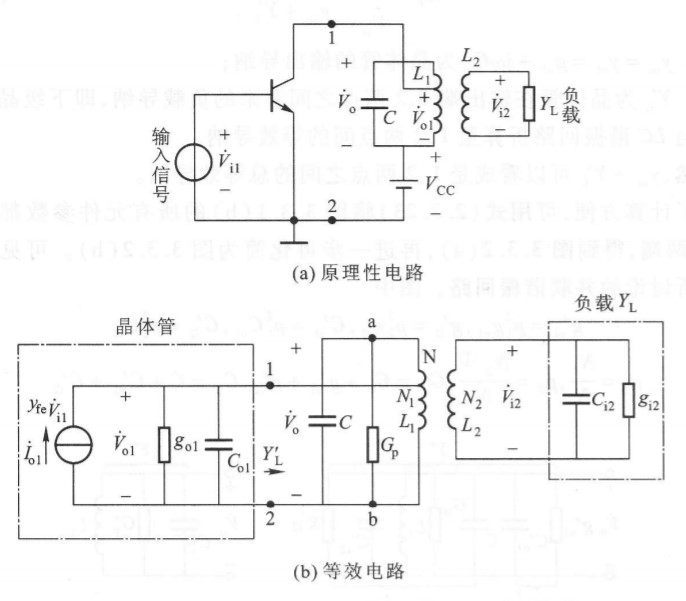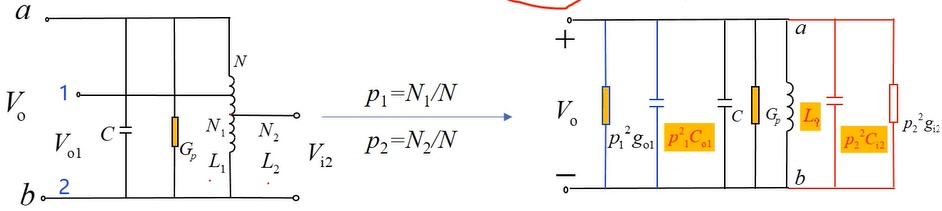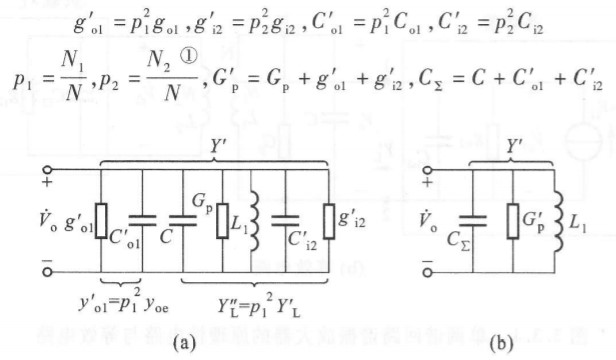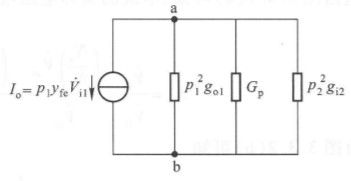# 单调谐回路谐振放大器1. $\dot{I}_{o1}=y_{fe}\dot{V}_{i1}$ 是晶体管放大作用的等效电流源
2. $g_{o1}, C_{o1}$ 为晶体管的输出导纳
3. $G_p=\frac{1}{R_p}$ 回路本身的损耗
4. $Y_L=g_{i2}+j\omega C_{i2}$ 负载本身的导纳（一般是下一级晶体管的输入导纳，所以用下标 $_i$）

## 电压增益（👇有损电压增益在功率增益部分👇）

## 功率增益m级：